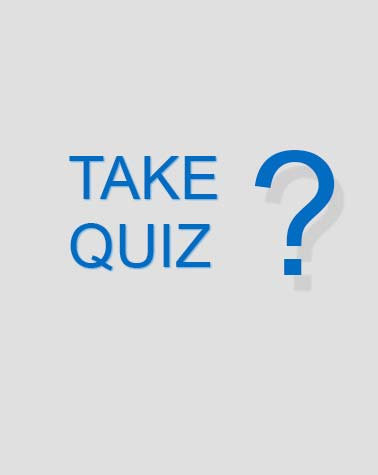# 3D152 Volume 1 UREs

69 Questions | Attempts: 391
ShareSettings3D152 Volume 1 UREs

• 1.
What is an example of analog-to-digital and digital-to-analog techniques?
• A.

Amplitude modulation (AM) and frequency modulation (FM).

• B.

Pulse code modulation (PCM) and frequency shift keying (FSK).

• C.

Phase modulation (PM) and quadrature phase shift keying (QPSK).

• D.

Pulse code modulation (PCM) and continuously variable slope delta (CVSD) modulation.

• 2.
What converts analog signal information into an analog carrier signal?
• A.

Delta modulation (DM).

• B.

Digital modulation.

• C.

Analog modulation.

• D.

• 3.
What converts analog signal information into a digital carrier signal?
• A.

Delta modulation (DM).

• B.

Analog modulation.

• C.

Digital modulation.

• D.

• 4.
The information-carrying components that occur on either side of a carrier are called
• A.

Sidebands.

• B.

Sine waves.

• C.

Frequency translators.

• D.

Modulating frequencies.

• 5.
Amplitude modulation (AM) requires a bandwidth that is
• A.

Twice that of the modulating signal.

• B.

Half that of the modulating signal.

• C.

Twice that of the carrier signal.

• D.

Half that of the carrier signal.

• 6.
The upper sideband in a single-sideband suppressed carrier (SSBSC) modulation is eliminated by
• A.

Filtering.

• B.

Detection.

• C.

Compression.

• D.

Companding.

• 7.
We can express the 6-to–1 improvement of the single-sideband suppressed carrier (SSBSC) modulation over conventional amplitude modulation (AM) as
• A.

2 decibels (dB) of improvement.

• B.

4 dB of improvement.

• C.

6 dB of improvement.

• D.

8 dB of improvement.

• 8.
The only basic waveform in nature is a
• A.

Carrier wave.

• B.

Pure sine wave.

• C.

Resultant wave.

• D.

Composite wave.

• 9.
A modulated waveform that contains a carrier plus two sidebands for each modulation frequency is a description of
• A.

A phase modulation (PM).

• B.

A pulse modulation.

• C.

A frequency modulation (FM).

• D.

An amplitude modulation (AM).

• 10.
The amount of deviation in a frequency modulation (FM) signal is directly proportional to the modulating signal’s
• A.

Phase.

• B.

Amplitude.

• C.

Frequency.

• D.

Amplitude and frequency.

• 11.
If 100 percent modulation is exceeded and there is distortion in the modulating signal, additional sidebands are generated. We identify these sidebands as what?
• A.

Transmitted intelligence.

• B.

Intensity of speech.

• C.

Composite waves.

• D.

Splatter.

• 12.
What is the main advantage of differential phase shift keying (DPSK)?
• A.

Carrier presence is required to detect changes.

• B.

It requires a less complex receiver than a basic phase shift keying (PSK) signal.

• C.

It requires a more complex receiver than a basic PSK signal.

• D.

The demodulator needs to detect changes in absolute phase values.

• 13.
Which of the following is not considered an analog modulation techniques?
• A.

Pulse-amplitude modulation (PAM).

• B.

Pulse-duration modulation (PDM).

• C.

Pulse-position modulation (PPM).

• D.

Pulse-code modulation (PCM).

• 14.
The amount of phase shift in a phase modulation (PM) signal is directly proportional to the
• A.

Modulation index.

• B.

Rate of phase shift.

• C.

Frequency of the modulating signal.

• D.

Amplitude of the modulating signal.

• 15.
What form of modulation is insensitive to signal variations?
• A.

Delta.

• B.

Frequency shift keying (FSK).

• C.

Pulse-amplitude modulation (PAM).

• D.

Binary-phase shift keying or bi-phase shift keying (BPSK).

• 16.
In quadrature-phase shift keying (QPSK) the radio frequency (RF) bandwidth for a quadriphase signal is what of that required by a bi-phase signal, given the same data rate.
• A.

Four times.

• B.

Double.

• C.

Half.

• D.

The same.

• 17.
The form of pulse modulation in which the pulse is varied within a set range of positions is known as
• A.

Pulse-amplitude modulation (PAM).

• B.

Pulse-duration modulation (PDM).

• C.

Pulse-position modulation (PPM).

• D.

Pulse-code modulation (PCM).

• 18.
Quantized analog modulation is actually
• A.

Pulse-amplitude modulation (PAM).

• B.

Pulse-duration modulation (PDM).

• C.

Less tolerant of noise than frequency modulation (FM).

• D.

Analog-to-digital conversion.

• 19.
How many bits per character of a 16-step pulse-code modulation (PCM) system are used to describe the quantized analog sample?
• A.

3.

• B.

4.

• C.

5.

• D.

8.

• 20.
The process of coding pulse code modulation (PCM) quantized pulses to make them a constant duration and amplitude is
• A.

Encoding.

• B.

Companding.

• C.

Uniform quantizing.

• D.

Nonuniform quantizing.

• 21.
In Time Division Multiplexing (TDM), the transmitting multiplexer will put a bit or a byte from each of the incoming lines into a specifically allocated what?
• A.

Channel.

• B.

Trunk.

• C.

Time slot.

• D.

Port.

• 22.
The two major types of frame structures are
• A.

Low speed and high speed.

• B.

High speed and EBCDIC.

• C.

CCITT and low speed.

• D.

EBCDIC and CCITT.

• 23.
The method of multiplexing the T1 multiplexer uses is
• A.

Frequency division.

• B.

Binary division.

• C.

Pulse division.

• D.

Time division.

• 24.
Wave-division multiplexing (WDM) creates the virtual channels for multiplexing them together for transmission by using different
• A.

Wavelengths or lambdas.

• B.

Wavelengths or gammas.

• C.

Frequencies or lambdas.

• D.

Frequencies or gammas.

• 25.
The most common wave-division multiplexing (WDM) system uses how many wavelengths?
• A.

One wavelength.

• B.

Two wavelengths.

• C.

Three wavelengths.

• D.

Four wavelengths.

## Related TopicsBack to top
×

Wait!
Here's an interesting quiz for you.